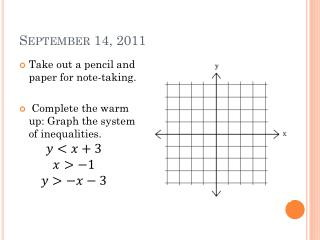# September 14, 2011 - PowerPoint PPT PresentationDownload PresentationSeptember 14, 2011

Download Presentation## September 14, 2011

- - - - - - - - - - - - - - - - - - - - - - - - - - - E N D - - - - - - - - - - - - - - - - - - - - - - - - - - -
##### Presentation Transcript

1. September 14, 2011 • Take out a pencil and paper for note-taking. • Complete the warm up: Graph the system of inequalities.

2. Linear Programming • Linear programming = a process of maximizing a linear objective function • Objective function = gives a quantity that is to be maximized (or minimized), and is subject to constraints • Constraints = the linear inequalities that form a system in a linear programming problem • Feasible region = the intersection of all the graphs of the constraints in a linear programming problem

3. Use linear programming to maximize profit • Toy wagons are made to sell at a craft fair. It takes 4 hours to make a small wagon and 6 hours to make a large wagon. The owner of the craft booth will make a profit of \$12 for a small wagon and \$20 for a large wagon. The craft booth owner has no more than 60 hours available to make wagons and wants to have at least 6 small wagons to sell. How many of each size should be made to maximize profit?

4. Use linear programming to maximize profit • Define variables. x = # of small wagons y = # of large wagons • Write objective function to show what you want to maximize or minimize. P = Profit P = 12x + 20y • Write constraints (inequalities). x ≥ 6 He wants to make at least 6 wagons. y ≥ 0 The # of large wagons cannot be negative. 4x + 6y ≤ 60 No more than 60 hours to work.

5. Use linear programming to maximize profit • Graph constraints. • Find vertices of shaded area. (6, 6) (6, 0) (15, 0)

6. Use linear programming to maximize profit • Plug vertices into objective function. At (6, 6): P = 12(6) + 20(6) = 192 At (6, 0): P = 12(6) + 20(0) = 72 At (15, 0): P = 12(15) + 20(0) = 180 • Answer the question in the problem. The owner can maximize profit by making 6 small wagons and 6 large wagons. His profit will be \$192.00. ← Maximum

7. Homework • Textbook pg. 32 #1-3, 5, 7, 8, 9